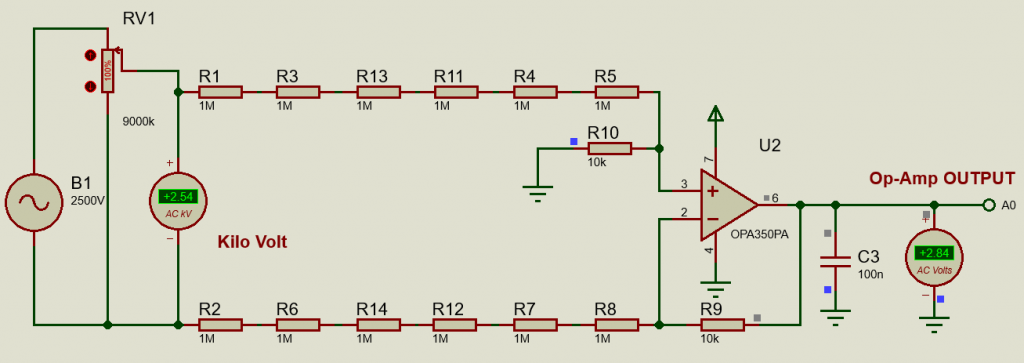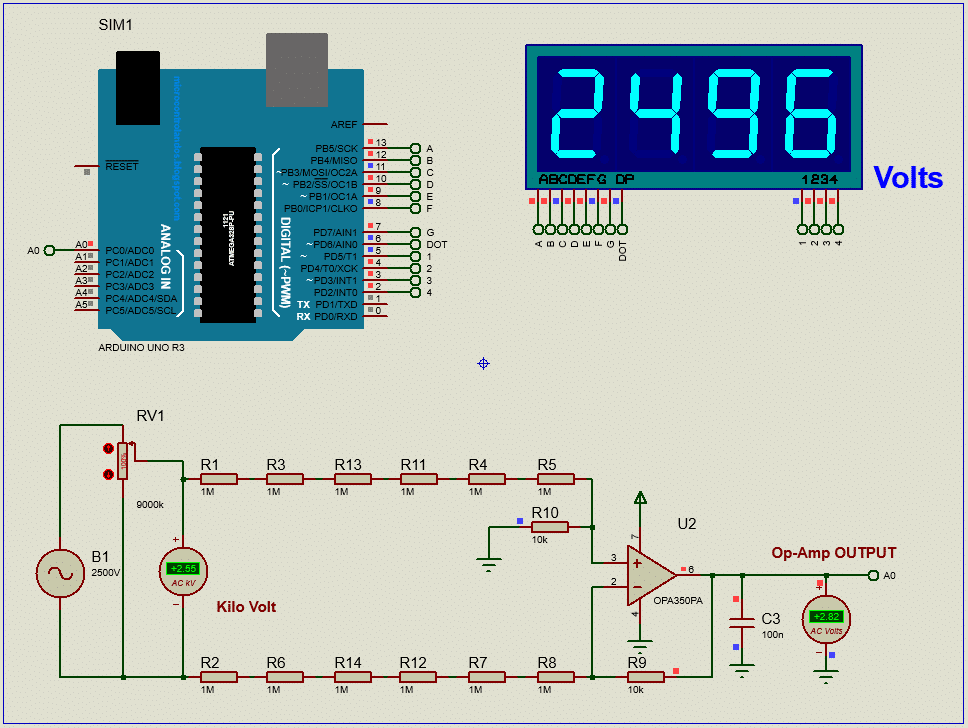# Making a High Voltage AC voltmeter with Arduino UNO and Op-Amp circuit, Measure 2500V !!!

In this article, we are going to learn how to make a high voltage AC voltmeter and we are going to measure 2500V !!!. Yes, we are measuring high voltage with our circuit. Lets do it…

Disclaimer: Electricity is always dangerous. Skill required to work with electricity. Do work with your own risk. Author will not be responsible for any misuse or harmful act. This website contents is also copyright protected. Anything copied and directly posted in your website and claiming it as yours is prohibited and nonsensical. Author published all the articles as opensource to help you making your project and learning purpose only. Learn and make one for yourself. If need any help feel free to ask the author. Author will be helpful to you. Thanks.

Table of Contents

## High voltage measurement technique:

Measuring high voltage is not safe for anyone. But if it is need to be done, then we must find a safe way to do the job. We should not measure high voltages keeping one terminal connected with the measuring instrument. Connecting with common terminal increases risk of getting electric shock, burn or fire. That is why, an isolation is required. Sometimes this isolation is done through high valued resistors, sometimes with opto-couplers. But in DC circuit, we can use high valued resistors in series to form an isolation. Adding an Op-Amp circuit, the process become more sensitive and accurate.

## Op-Amp based high voltage measurement circuit:Op-Amp circuit for High voltage AC measurement

Here in this circuit, we use an Op-Amp and several resistors to make a floating or isolated voltage measuring circuit. The circuit simply measuring the voltage difference between two points. This differential amplifier circuit can be used in many types of measuring circuit where we can measure voltage differences of two points. Simple configuration and simple calculation.

Here, Gain = R9/(R2+R6+R7+R8)

## Circuit diagram:Circuit diagram

## Arduino Coding:

Before reading the code, please check my other article about this, “How to make an AC voltmeter using Arduino UNO and 4×1 multiplexed Seven Segment display“. Then it will be easy to understand.

```#include <TimerOne.h>
const char segment_pins[] = {13, 12, 11, 10, 9, 8, 7, 6}; //segments a to g
const char digit_pins[] = {5, 4, 3, 2}; // 4digits
const int digit_number = 4;//for 4 digit segment
byte dot_position;//set dot position from right side.
bool dot_point = false; // having dot or not, true = have dot, false = no dot.

const int segment_array = // for common cathode segment only
{
{HIGH, HIGH, HIGH, HIGH, HIGH, HIGH, LOW, LOW}, //0
{LOW, HIGH, HIGH, LOW, LOW, LOW, LOW, LOW},     //1
{HIGH, HIGH, LOW, HIGH, HIGH, LOW, HIGH, LOW},  //2
{HIGH, HIGH, HIGH, HIGH, LOW, LOW, HIGH, LOW},  //3
{LOW, HIGH, HIGH, LOW, LOW, HIGH, HIGH, LOW},   //4
{HIGH, LOW, HIGH, HIGH, LOW, HIGH, HIGH, LOW},  //5
{HIGH, LOW, HIGH, HIGH, HIGH, HIGH, HIGH, LOW}, //6
{HIGH, HIGH, HIGH, LOW, LOW, LOW, LOW, LOW},    //7
{HIGH, HIGH, HIGH, HIGH, HIGH, HIGH, HIGH, LOW},//8
{HIGH, HIGH, HIGH, HIGH, LOW, HIGH, HIGH, LOW}, //9
};

void int_sev_segment()
{
for (int k = 0; k < 8; k++)
{
pinMode(segment_pins[k], OUTPUT);//I/O settings
}
for (int k = 0; k < digit_number; k++)
{
pinMode(digit_pins[k], OUTPUT);//I/O settings
}
for (int k = 0; k < 8; k++)
{
digitalWrite(segment_pins[k], LOW);//keep low
}
for (int k = 0; k < digit_number; k++)
{
digitalWrite(digit_pins[k], HIGH);//keep high = disable segments (CC)
}
Timer1.initialize(5000);//5000us = 5ms
Timer1.attachInterrupt(display_segment);
}

char digits;
void display_7segment(int number, byte dot)
{
digits = number / 1000u;//extract 1000th digit
digits = (number / 100u) % 10u;//extract 100th digit
digits = (number / 10u) % 10u;//extract 10th digit
digits = (number / 1u) % 10u;//extract 1st digit
dot_position = dot;
}

int digit_position = 0;
void display_segment(void) // called periodically using timer interrupt
{
for (int k = 0; k < digit_number; k++)
{
digitalWrite(digit_pins[k], HIGH);//reset digit pins
}
if (digit_position > 3)digit_position = 0;

for (int k = 0; k < 8; k++) //print the a to g segment pins
{
digitalWrite(segment_pins[k], segment_array[digits[digit_position]][k]);
if (digit_position == dot_position && dot_point == true)
{
digitalWrite(segment_pins, HIGH);//print dot point
}
}

if (digit_position == 3)
{
digitalWrite(digit_pins, LOW);
digitalWrite(digit_pins, HIGH);
digitalWrite(digit_pins, HIGH);
digitalWrite(digit_pins, HIGH);
}
else if (digit_position == 2)
{
digitalWrite(digit_pins, HIGH);
digitalWrite(digit_pins, LOW);
digitalWrite(digit_pins, HIGH);
digitalWrite(digit_pins, HIGH);
}
else if (digit_position == 1)
{
digitalWrite(digit_pins, HIGH);
digitalWrite(digit_pins, HIGH);
digitalWrite(digit_pins, LOW);
digitalWrite(digit_pins, HIGH);
}
else if (digit_position == 0)
{
digitalWrite(digit_pins, HIGH);
digitalWrite(digit_pins, HIGH);
digitalWrite(digit_pins, HIGH);
digitalWrite(digit_pins, LOW);
}
digit_position++;
}

long ac_voltage = 0;
unsigned int temp, maxpoint;
void setup()
{
int_sev_segment();//initialize seven segment program
}

void loop()
{
ac_voltage = 0; //clear previous result
temp = 0;
maxpoint = 0;
for (int i = 0; i < 10; i++)
{
for(int m=0;m<500;m++)
{
if(temp = analogRead(A0);temp>maxpoint)
{
maxpoint = temp;
}
}
ac_voltage += maxpoint*2.455;
}
ac_voltage /= 10; //get average value

display_7segment(ac_voltage, 2); //display value of cnt, dot in position 1 from right side

}

// end```

## Conclusion:

In this project, you learned how to make an AC voltmeter that can measure 2500V (!!!) with Arduino and Op-Amp circuit. The result is displayed in multiplexed Seven segment display. We tested the voltmeter in simulation. This project is now ready for practical use.

Kindly select voltage divider resistors carefully to measure higher voltage. Proper selection can increase the resolution of our voltmeter.

But keep in mind that electricity is always dangerous. Do work on your own risk.

I hope, you enjoyed this project and now you can make one for yourself. If you are stuck in middle and need help, just ask. I’ll try my best to help you. Thank you, Enjoy!

#### MKDas

Mithun K. Das; B.Sc. in EEE from KUET. Blog: https://labprojectsbd.com

### 4 Comments#### djalltra · October 20, 2020 at 11:11 am

can you please make the mikroc version#### djallra · October 21, 2020 at 10:51 am

i notice you did not connect a 2.5v offset to this circuit why so?